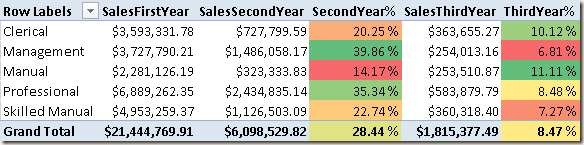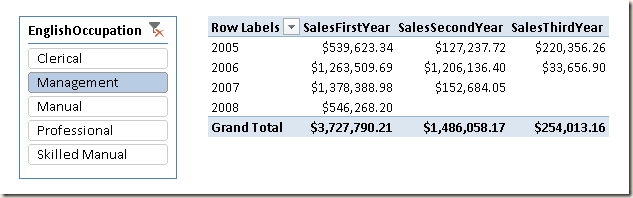Consider the classical Adventure Works scenario. You want to see, for each Occupation, the total amount of sales made on the first three years, considering for each customer the date of the first order.As you see in the PivotTable below, Management and Professional are the two occupations that spends more in the second year, whereas Clerical and Manual occupations  produce higher revenues in the third year.The calculation must also consider a possible selection of dates. For example, you might want to see the amount of sales year by year made by customers in their first, second and third year of purchases.In order to implement the calculation, you need to establish the date of the first order for each customer. Even if you could implement this calculation as a measure, for performance reasons it is a good idea persisting that value in a calculated column. You can implement the FirstOrder calculated column in the Customer table using the following expression:

```FirstOrder =
CALCULATE (
MIN ( 'Internet Sales'[OrderDate] ),
ALL ( 'Date' )
)```

You create the measure for the sales made to a customer in the first year since its first order by using the following expression:

```SalesFirstYear:=
SUMX(
FILTER (
VALUES ( Customer[FirstOrder] ),
CONTAINS ( 'Date', 'Date'[Date], Customer[FirstOrder] )
),
CALCULATE (
SUM ( 'Internet Sales'[SalesAmount] ),
DATESINPERIOD (
'Date'[Date],
Customer[FirstOrder],
12,
MONTH
)
)
)```

The calculation should be done customer by customer. For this reason the external SUMX performs an iteration considering only those customers that have their first order date within the range of dates currently selected in the PivotTable. In order to optimize the performance, the FILTER use the list of distinct FirstOrder dates, because that number is usually lower than the number of customers and the following expression used by SUMX can be executed only once for all the customers that have the same FirstOrder date value. The CALCULATE applies a filter over dates considering exactly one year starting on the given FirstOrder date.

You implement the measure for the second and third year by wrapping the DATESINPERIOD result into a DATEADD call that moves the range of dates forward of the required number of years.

```SalesSecondYear :=
SUMX(
FILTER (
VALUES ( Customer[FirstOrder] ),
CONTAINS ( 'Date', 'Date'[Date], Customer[FirstOrder] )
),
CALCULATE (
SUM ( 'Internet Sales'[SalesAmount] ),
DATESINPERIOD (
'Date'[Date],
Customer[FirstOrder],
12,
MONTH
),
1,
YEAR
)
)
)```

```SalesThirdYear :=
SUMX(
FILTER (
VALUES ( Customer[FirstOrder] ),
CONTAINS ( 'Date', 'Date'[Date], Customer[FirstOrder] )
),
CALCULATE (
SUM ( 'Internet Sales'[SalesAmount] ),
DATESINPERIOD (
'Date'[Date],
Customer[FirstOrder],
12,
MONTH
),
2,
YEAR
)
)
)```

The presence of a CALCULATE in an iteration such as SUMX is always a possible bottleneck of a DAX expression. In this case this approach is necessary, because of the calculation you need, but you can reduce the performance issue by minimizing the number of rows iterated by SUMX, finding the minimum granularity that produces the same result.

SUMXReturns the sum of an expression evaluated for each row in a table.

`SUMX ( <Table>, <Expression> )`

FILTERReturns a table that has been filtered.

`FILTER ( <Table>, <FilterExpression> )`

CALCULATEContext transition

Evaluates an expression in a context modified by filters.

`CALCULATE ( <Expression> [, <Filter> [, <Filter> [, … ] ] ] )`

DATESINPERIODReturns the dates from the given period.

`DATESINPERIOD ( <Dates>, <StartDate>, <NumberOfIntervals>, <Interval> )`

DATEADDContext transition

Moves the given set of dates by a specified interval.

`DATEADD ( <Dates>, <NumberOfIntervals>, <Interval> )`